NetworkX

#### Previous topic

degree_pearson_correlation_coefficient

#### Next topic

average_degree_connectivity

# average_neighbor_degree¶

average_neighbor_degree(G, source='out', target='out', nodes=None, weight=None)

Returns the average degree of the neighborhood of each node.

The average degree of a nodeis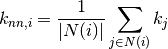where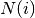are the neighbors of nodeand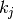is the degree of node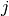which belongs to. For weighted graphs, an analogous measure can be defined [R133],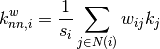where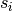is the weighted degree of node,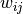is the weight of the edge that linksandandare the neighbors of node.

Parameters : G : NetworkX graph source : string (“in”|”out”) Directed graphs only. Use “in”- or “out”-degree for source node. target : string (“in”|”out”) Directed graphs only. Use “in”- or “out”-degree for target node. nodes : list or iterable, optional Compute neighbor degree for specified nodes. The default is all nodes in the graph. weight : string or None, optional (default=None) The edge attribute that holds the numerical value used as a weight. If None, then each edge has weight 1. d: dict : A dictionary keyed by node with average neighbors degree value.

Notes

For directed graphs you can also specify in-degree or out-degree by passing keyword arguments.

References

 [R133] (1, 2) A. Barrat, M. Barthélemy, R. Pastor-Satorras, and A. Vespignani, “The architecture of complex weighted networks”. PNAS 101 (11): 3747–3752 (2004).

Examples

>>> G=nx.path_graph(4)
>>> G.edge['weight'] = 5
>>> G.edge['weight'] = 3

>>> nx.average_neighbor_degree(G)
{0: 2.0, 1: 1.5, 2: 1.5, 3: 2.0}
>>> nx.average_neighbor_degree(G, weight='weight')
{0: 2.0, 1: 1.1666666666666667, 2: 1.25, 3: 2.0}

>>> G=nx.DiGraph()
>>> G.add_path([0,1,2,3])
>>> nx.average_neighbor_degree(G, source='in', target='in')
{0: 1.0, 1: 1.0, 2: 1.0, 3: 0.0}

>>> nx.average_neighbor_degree(G, source='out', target='out')
{0: 1.0, 1: 1.0, 2: 0.0, 3: 0.0}